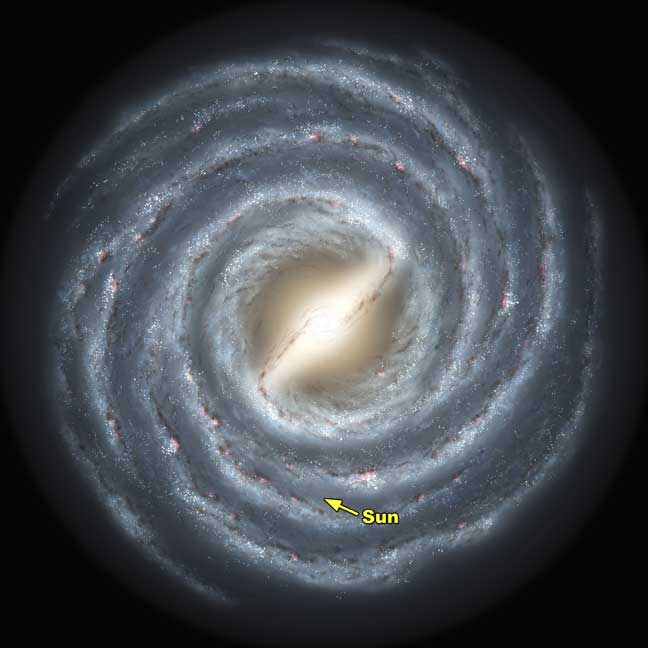# At What Speed Does The Milky Way Move Through Space?

## 3 Answers

Anonymous answered
If the milky way is moving through space, are all other galaxies surrounding the milky way moving at exactly the same rate? It seems that if the milky way is moving through space, the environment outside the milky way would change as it moves through different areas of space. Does this happen?
thanked the writer.
Chris Lee answered
Astronomers think the Milky Way is traveling at around 600 km per
second (which is 1,342,161 miles per hour) relative to the locations of other galaxies.

Recent estimates range from 130 km a second to 1,000 km a second.

If the Galaxy is moving at 600 km/s, Earth travels 51.84 million km per
day (32,211,882 miles, or more than 18.9 billion km per year (1.17439155 × 1010 miles).

Sources: Wikipedia -  Milky Way,  public.iastate.eduthanked the writer.
Anonymous answered
1.3 million miles per hour depending on where you are. Galaxies are moving away from the earth. The rate of speed has been proven to be increasing.
thanked the writer.

## Answer Question